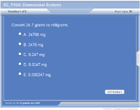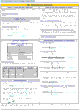How to Learn in 24 Hours?

 Need Help? M-F: 9am-5pm(PST): Toll-Free: (877) RAPID-10 or 1-877-727-4310 24/7 Online Technical Support: The Rapid Support Center vip@rapidlearningcenter.com Secure Online Order:Need Proof? Testimonials by Our Users

 Rapid Learning Courses: MCAT in 24 Hours (2021-22) USMLE in 24 Hours (Boards) Chemistry in 24 Hours Biology in 24 Hours Physics in 24 Hours Mathematics in 24 Hours Psychology in 24 Hours SAT in 24 Hours ACT in 24 Hours AP in 24 Hours CLEP in 24 Hours DAT in 24 Hours (Dental) OAT in 24 Hours (Optometry) PCAT in 24 Hours (Pharmacy) Nursing Entrance Exams Certification in 24 Hours eBook - Survival Kits Audiobooks (MP3)

 Tell-A-Friend: Have friends taking science and math courses too? Tell them about our rapid learning system.Home »  Chemistry »  SAT Chemistry

Dimensional Analysis

Topic Review on "Title":

Dimensional analysis is used to convert units.

The Principle Behind Dimensional Analysis
The guiding principle of dimensional analysis is that you can multiply anything by “1” without changing the meaning.  An equality set into a fraction formation = 1.  For example, if x = y, then x/y = 1 and  y/x = 1.  Therefore, the equalities can be set into fractions and multiplied to convert units.

Another concept necessary to understanding dimensional analysis is that units that are on the top and bottom of an expression cancel out.

Equalities Commonly used in Dimensional Analysis
Several equalities are used often in chemistry.

 Equalities commonly used in chemistry 4.18 J 1.00 cal 1 Å 10‑10 m 1 cm3 1 mL 1 dm3 1 L 1 in 2.54 cm 1 kg 2.2 lb 1 atm 101.3 kPa 1 atm 760 mm Hg 1 mole 6.02 ´ 1023 pieces

Metric prefixes are also used to form equalities between different metric units.

Dimensional Analysis
To work dimensional analysis problems:

• Write your known down on the left side
• Write down “=__________ [desired unit]” at the right side
• Identify equalities that will get you from the known information to the desired unit.  If there is no equality that involves both the known and unknown, you’ll have to find more than one to more than one step.
• Arrange the equalities into a fractional form so that the known unit will cancel out and the desired unit will be left.
• Multiply across the top of the expression and divide numbers on the bottom.

Rapid Study Kit for "Title":
 Flash Movie Flash Game Flash Card Core Concept Tutorial Problem Solving Drill Review Cheat Sheet"Title" Tutorial Summary : Dimensional analysis is a technique for converting units based upon equalities.  The technique is the basis for stoichiometry—a key chemistry calculation—presented later in the series.

 Tutorial Features: Specific Tutorial Features: Problem-solving techniques are used to work out the example problems. Series Features: Concept map showing inter-connections of new concepts in this tutorial and those previously introduced. Definition slides introduce terms as they are needed. Visual representation of concepts Animated examples—worked out step by step A concise summary is given at the conclusion of the tutorial.

 "Title" Topic List: What is dimensional analysis? What is the principle behind dimensional analysis? What equalities are used commonly in chemistry? How is dimensional analysis performed? How is dimensional analysis used in chemistry?

See all 24 lessons in college chemistry, including concept tutorials, problem drills and cheat sheets:
Teach Yourself SAT Chemistry Visually in 24 Hours